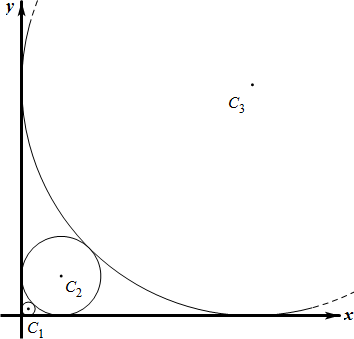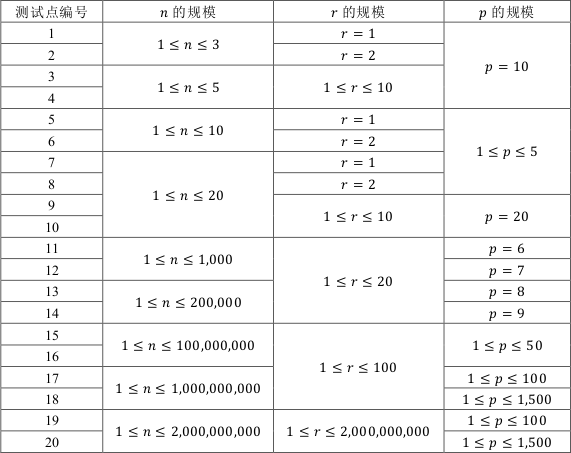# YZOJ P4587 斐波那契数列

• ### 样例输出

Source： BZOJ 5104…

# YZOJ P3033 背包

• ### 题目描述

（即每种物品按照 $$1,2,3, \cdots ,b_i$$ 编号的方案数和不编号的方案数。）

# YZOJ P2697 画圆

• ### 题目描述

$$Alkri$$ 想在平面直角坐标系的第一象限中依次画 $$n$$ 个与两坐标轴均相切的圆，其中，第 $$1$$ 个圆的半径为 $$r$$，之后的每个圆都比上一个圆大，且与上一个圆相切，也就是说，对所有整数 $$2 \leq i \leq n$$，第 $$i$$ 个圆的半径大于第 $$i-1$$ 个圆的半径且与第 $$i-1$$个圆相切。• ### 数据规模与约定…